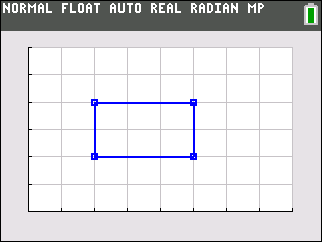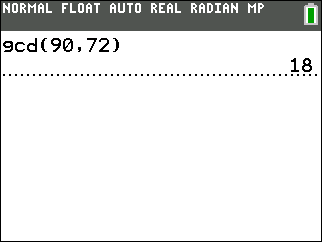# Activities

••• ##### Subject Area

• Math: Middle Grades Math: Geometry
• Math: Middle Grades Math: Problem Solving

• ##### Author6-8

45 Minutes

• ##### Device
• TI-83 Plus Family
• TI-84 Plus
• TI-84 Plus Silver Edition
•TI-84 Plus C Silver Edition
•TI-84 Plus CE

TI Connect™

## Comparing Area of Rectangles#### Activity Overview

In this activity, students discover the relationship between a change in the dimensions of a rectangle and the change in the corresponding area.

#### Key Steps

•In this activity, students determine the dimensions and area of given rectangles. They will determine a pattern between the sides lengths and the resulting area. Students will use what they learned to solve 3 word problems.

•In the third problem, students are to find the dimensions of two rectangles that share one side, given the area of both rectangles. They will use the gcd command to find the greatest common factor of the two areas.